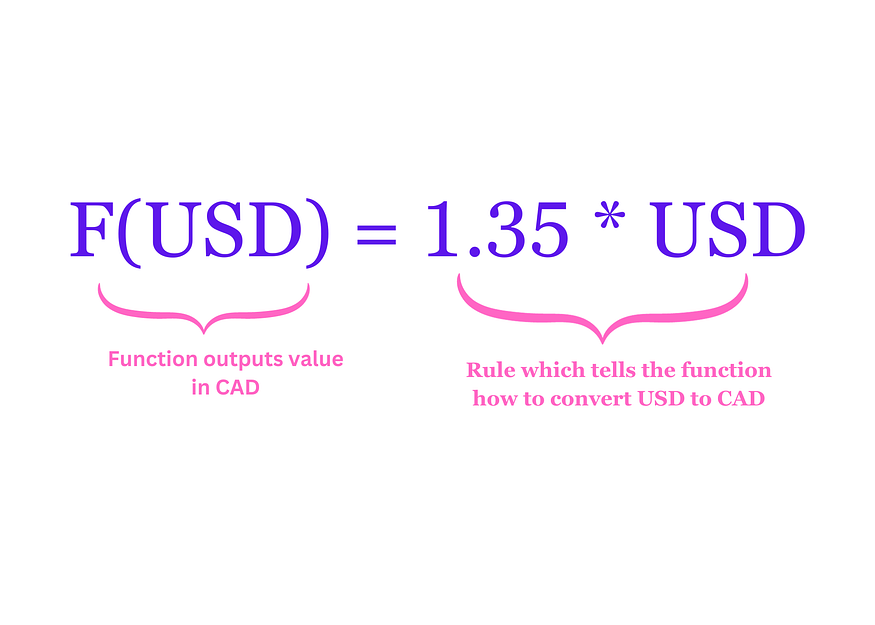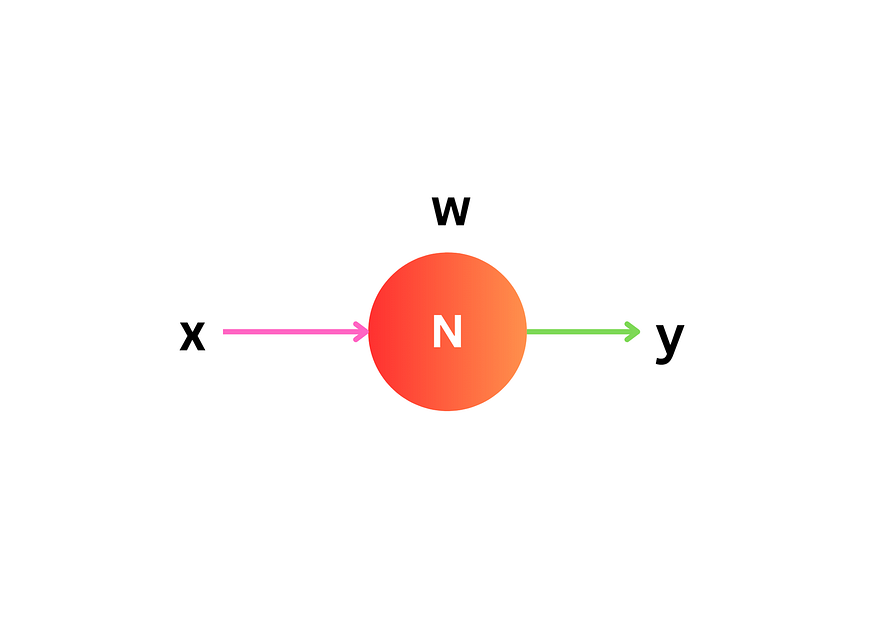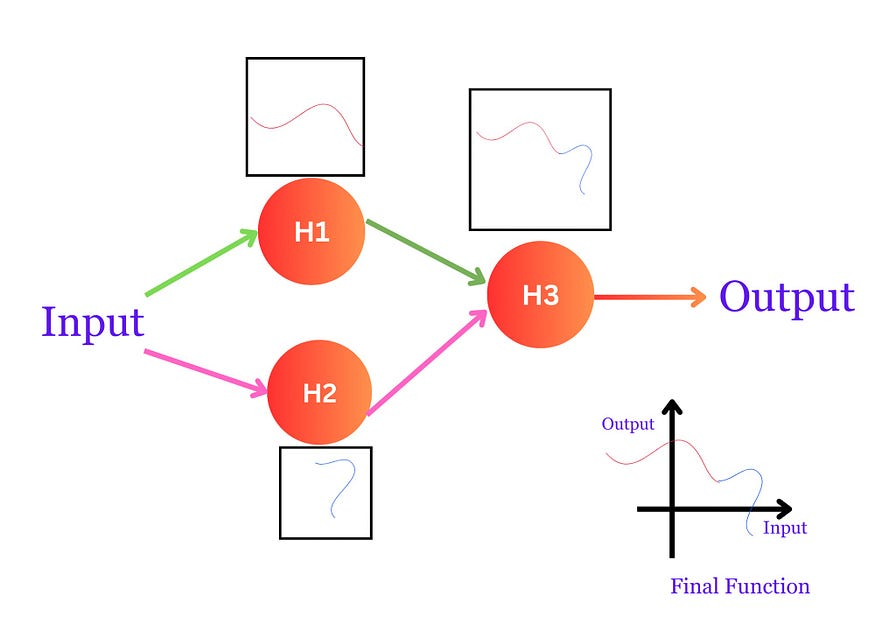Master LLMs with our FREE course in collaboration with Activeloop & Intel Disruptor Initiative. Join now!

## Publication# The One Thing You Need To Truly Understand Neural Networks is to Understand …

Last Updated on November 5, 2023 by Editorial Team

#### Author(s): Nandini Tengli

Originally published on Towards AI.

Neural Networks can learn almost anything.

From learning to differentiate between Dogs and Cats to learning to be THE go-to chatbot for 100s of millions of people. Neural Networks seem like magic.

What if I told you that all you need to understand Neural Networks is to know what a function is?

A function, in this blog, is going to be simply something to which, when you give an input, it spits out an output according to a set of rules.

For example, the function to convert US dollars to Canadian dollars would take the dollar amount in USD as input and spit out the Canadian Dollar amount by multiplying the input by 1.35.

*note — the conversion rate (1.35) will fluctuate based on the Forex market, this is just the rate at the time of writing!

To understand Neural Networks better, let us start at the level of a ‘Neuron’ — the building block of a Neural Network.

Think of a Neuron as simply a function — something to which when you give an input, it spits out a meaningful output. Except, the job of a Neuron is to not only apply the rule but also to come up with it.

We supply it with a lot of data — a set of inputs and the corresponding outputs. The neuron then “learns” or comes up with a rule that maps the inputs to the outputs. Now, when we give it a totally new input, it is able to give us an output.

This is the point of Machine Learning.

For simple data, something linear, for example, it is easy for us to come up with the “rule” mapping the inputs to the outputs on our own. It is just about calculating the ‘inverse function’, something you may have done in high school.

But when the data is complex and when the data is massive — like when we want to be able to map the entire internet — we use machine learning models like neural networks to come up with the rules of how inputs relate to outputs.

Let us look at a very simple depiction of a neural network neuron below:Figure 2: A simple Neuron accepting inputs (x) and producing outputs (y) based on w

The neuron takes the input x and gives us an output based on the rule w. Compare w to the conversion factor in the USD → CAD example.

A Neuron first takes in a set of inputs (Xs — ex. many values in USD) and a set of corresponding outputs (Ys — corresponding values in CAD). It then figures out the rules — the W for this data — which maps the inputs to the outputs (e.g., a rule that maps our value in USD to Canadian dollars)

Now, when you give it a USD input, it will provide you with an output in CAD!

A bunch of neurons make up a neural network. Think of each neuron as working on a small part of a big, complicated function. We need multiple neurons because data in the real world is complicated and rarely fits a neat linear or polynomial function. If we take our example of converting USD to CAD, the conversion rate depends on multiple factors like inflation, debt of a country, political climate, etc., making coming up with the conversion rate complex.

You can think of it like it is each neuron's job to develop the rules for a part of the larger, more complex function. So as a whole, the neural network comes up with the “rule” that maps the inputs onto the outputs. This makes neural networks essentially layers of ‘functions’ where we feed in inputs to each layer and output from that layer feeds it as inputs to the next layer and so on.

The picture below shows this in action!Figure 3: A conceptional picture of a Neural network coming up with the rule

**Note: This image should only be used for a conceptual understanding!

When we use a trained Neural Network, i.e., when we give it an input expecting an output to a neural network, neurons inside the network take the input and give us an output based on the rules that it has already figured out for that type of data.

A trained neural network is simply a neural network that knows the ‘rules’ for the type of data you are going to give it. It is able to provide predictions and outputs when you give it inputs. For example, ChatGPT — or GPT 4 knows the rules of language. This is why when you say hello, it says hi (or something similar) back.

In order to get to this stage, the neural network needs to first be ‘trained’.

Suppose we had some Foreign Exchange data: Dollar amounts in USD and their equivalents in CAD. But suppose we did not know the conversion rate (let’s say we didn’t know that it was 1.35 from above)

So, we feed this set of inputs (dollar amounts in USD) and the outputs (Canadian dollar amounts) into the neural network.

Using this set of inputs and outputs, the Neural Network would systematically try different values for the conversion rate, and try to come up with the best value for the conversion rate. This is what goes on during training.

It comes up with a value — let’s say it comes up with 10. It applies the rule on the set of inputs provided. It then compares its set of outputs to the set of outputs we provide to the network. It realizes that it is wrong. It then tries a different value, depending on how wrong it was. Since it picked 10, which is too high for the conversion rate, its next guess will be lower — say 5.

This process of trial and error is informed by a system inside the neural network. There are a set of calculations — ex. mean squared error is what the neural network can do to figure out what the next best guess would be.

It keeps trying different values, evaluating them, and trying new values until it minimizes the ‘error’ — the discrepancy between the outputs it is able to calculate based on the rules it came up with and the outputs that we have given it. When the discrepancy is the least, its training is complete, and it has figured out a ‘good-enough’ rule for the set of data.

Now, when we give it a new USD dollar amount, the NN would be able to tell us the CAD equivalent even if the amount we gave it was not part of the input dataset!

It has figured out the rule!

This process of figuring out the rule is the learning in Machine Learning.

More Resources:

Join thousands of data leaders on the AI newsletter. Join over 80,000 subscribers and keep up to date with the latest developments in AI. From research to projects and ideas. If you are building an AI startup, an AI-related product, or a service, we invite you to consider becoming a sponsor.

Published via Towards AI